GATE  >  Production And Industrial Engineering - PI 2015 GATE Paper (Practice Test)

# Production And Industrial Engineering - PI 2015 GATE Paper (Practice Test) - GATE

Test Description

## 65 Questions MCQ Test GATE Past Year Papers for Practice (All Branches) - Production And Industrial Engineering - PI 2015 GATE Paper (Practice Test)

Production And Industrial Engineering - PI 2015 GATE Paper (Practice Test) for GATE 2023 is part of GATE Past Year Papers for Practice (All Branches) preparation. The Production And Industrial Engineering - PI 2015 GATE Paper (Practice Test) questions and answers have been prepared according to the GATE exam syllabus.The Production And Industrial Engineering - PI 2015 GATE Paper (Practice Test) MCQs are made for GATE 2023 Exam. Find important definitions, questions, notes, meanings, examples, exercises, MCQs and online tests for Production And Industrial Engineering - PI 2015 GATE Paper (Practice Test) below.
Solutions of Production And Industrial Engineering - PI 2015 GATE Paper (Practice Test) questions in English are available as part of our GATE Past Year Papers for Practice (All Branches) for GATE & Production And Industrial Engineering - PI 2015 GATE Paper (Practice Test) solutions in Hindi for GATE Past Year Papers for Practice (All Branches) course. Download more important topics, notes, lectures and mock test series for GATE Exam by signing up for free. Attempt Production And Industrial Engineering - PI 2015 GATE Paper (Practice Test) | 65 questions in 180 minutes | Mock test for GATE preparation | Free important questions MCQ to study GATE Past Year Papers for Practice (All Branches) for GATE Exam | Download free PDF with solutions
 1 Crore+ students have signed up on EduRev. Have you?
Production And Industrial Engineering - PI 2015 GATE Paper (Practice Test) - Question 1

### Q 1  - Q 5 carry one mark. Choose the appropriate word/phrase, out of the four options given below, to complete the following sentence: Apparent lifelessness ________ dormant life.

Production And Industrial Engineering - PI 2015 GATE Paper (Practice Test) - Question 2

### Fill in the blank with the correct idiom/phrase. That boy from the town was a ________ in the sleepy village.

Production And Industrial Engineering - PI 2015 GATE Paper (Practice Test) - Question 3

### Choose the statement where underlined word is used correctly.

Production And Industrial Engineering - PI 2015 GATE Paper (Practice Test) - Question 4

Tanya is older than Eric.

Cliff is older than Tanya.

Eric is older than Cliff.

If the first two statements are true, then the third statement is

Production And Industrial Engineering - PI 2015 GATE Paper (Practice Test) - Question 5

Five teams have to compete in a league, with every team playing every other team exactly once, before going to the next round. How many matches will have to be held to complete the league round of matches.

Production And Industrial Engineering - PI 2015 GATE Paper (Practice Test) - Question 6

Q 6  - Q 10 carry one mark.

Select the appropriate option in place of underlined part of the sentence.

"Increased productivity necessary" reflects greater efforts made by the employees.

Production And Industrial Engineering - PI 2015 GATE Paper (Practice Test) - Question 7

Given below are two statements followed by two conclusions. Assuming these statements to be true, decide which one logically follows.

Statements:

1. No Manager is a leader.

Conclusions:

1. No manager is an executive.

2. No executive is a manager.

*Answer can only contain numeric values
Production And Industrial Engineering - PI 2015 GATE Paper (Practice Test) - Question 8

In the given figure angle Q is a right angle, PS:QS = 3:1, RT:QT = 5:2 and PU:UR = 1:1. If area of triangle QTS is 20 cm2, then the area of triangle PQR in cm2 is _______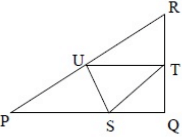(Important - Enter only the numerical value in the answer)

Production And Industrial Engineering - PI 2015 GATE Paper (Practice Test) - Question 9

Right triangle PQR is to be constructed in the xy-plane so that the right angle is at P and line PR is parallel to x-axis. The x and y coordinates of P,Q and R are to be integers that satisfy the inequalities :

-4 < x< 5 and 6 < y < 16. How many different triangles could be constructed with these properties?

Production And Industrial Engineering - PI 2015 GATE Paper (Practice Test) - Question 10

A coin is tossed thrice. Let X be the event that head occurs in each of the two tosses. Let Y be the event that a tail occurs on the third toss. Let Z be the event that two tails occur in three tosses. Based on the above information, which one of the following statements is TRUE?

Production And Industrial Engineering - PI 2015 GATE Paper (Practice Test) - Question 11

Q. 11 – Q. 35 carry one mark each.
Q. In numerical integration using Simpson’s rule, the approximating function in the interval is a

Production And Industrial Engineering - PI 2015 GATE Paper (Practice Test) - Question 12

If a constant force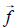applied on an object P, displaces it by a distance ,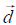inclined at an angle θ to the direction of force,then the work done by the forceis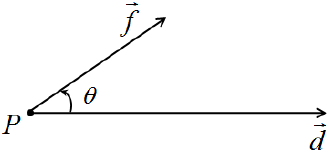Production And Industrial Engineering - PI 2015 GATE Paper (Practice Test) - Question 13

A product is an assembly of 5 different components. The product can be sequentially assembled in two possible ways. If the 5 components are placed in a box and these are drawn at random from the box, then the probability of getting a correct sequence is

Production And Industrial Engineering - PI 2015 GATE Paper (Practice Test) - Question 14

The function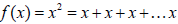times, is defined

Production And Industrial Engineering - PI 2015 GATE Paper (Practice Test) - Question 15

The room-temperature stress (σ)-strain (ε) curves of four materials P, Q, R, and S are shown in the figure below. The material that behaves as a rigid perfectly plastic material is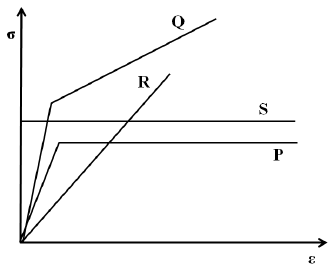*Answer can only contain numeric values
Production And Industrial Engineering - PI 2015 GATE Paper (Practice Test) - Question 16

The true stress at fracture of a tensile tested specimen, having an initial diameter of 13 mm, is 700 MPa. If the diameter of specimen at fracture is 10 mm, then the engineering stress, in MPa, at fracture is _________________.

(Important - Enter only the numerical value in the answer)

*Answer can only contain numeric values
Production And Industrial Engineering - PI 2015 GATE Paper (Practice Test) - Question 17

If the principal stress values are 120 MPa, – 50 MPa and 10 MPa in a given state of stress, then maximum shear stress in the material, in MPa, is ___________________.

(Important - Enter only the numerical value in the answer)

Production And Industrial Engineering - PI 2015 GATE Paper (Practice Test) - Question 18

Match the items in the first column to their functions in the second column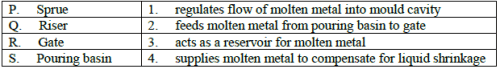Production And Industrial Engineering - PI 2015 GATE Paper (Practice Test) - Question 19

In rolling of a flat strip, the relative velocity of strip with respect to the roller is

*Answer can only contain numeric values
Production And Industrial Engineering - PI 2015 GATE Paper (Practice Test) - Question 20

The maximum reduction per pass during wire drawing of an aluminum alloy ignoring friction and redundant work is 77%. The strain hardening exponent of the material is ____________.

(Important - Enter only the numerical value in the answer)

Production And Industrial Engineering - PI 2015 GATE Paper (Practice Test) - Question 21

Built-up edge formation decreases under the conditions listed below EXCEPT

Production And Industrial Engineering - PI 2015 GATE Paper (Practice Test) - Question 22

During turning of mild steel work material, the maximum temperature is observed at

Production And Industrial Engineering - PI 2015 GATE Paper (Practice Test) - Question 23

Which one of the following statements related to grinding process is INCORRECT?

Production And Industrial Engineering - PI 2015 GATE Paper (Practice Test) - Question 24

For an assembly made of n components, the dimensions on each component i follow a normal distribution and have tolerance Ti. Overall dimension of the assembly is La with tolerance Ta. The relationship between Ta and Ti is

Production And Industrial Engineering - PI 2015 GATE Paper (Practice Test) - Question 25

Which of the following DO NOT influence the material removal rate in Electrical Discharge Machining process?

(i) Hardness of work piece material

(ii) Melting temperature of work piece material

(iii)Hardness of tool material

(iv) Discharge current and frequency

Production And Industrial Engineering - PI 2015 GATE Paper (Practice Test) - Question 26

In Computer Aided Process Planning, determination of process sequence for manufacture of any part design without predefined standard plans is known as

Production And Industrial Engineering - PI 2015 GATE Paper (Practice Test) - Question 27

The angle of a twist drill that determines its rake angle is

Production And Industrial Engineering - PI 2015 GATE Paper (Practice Test) - Question 28

A line balancing problem is solved in the context of

Production And Industrial Engineering - PI 2015 GATE Paper (Practice Test) - Question 29

Solution to the balanced assignment problem is binary due to

Production And Industrial Engineering - PI 2015 GATE Paper (Practice Test) - Question 30

Material Requirements Planning DOES NOT include

Production And Industrial Engineering - PI 2015 GATE Paper (Practice Test) - Question 31

Ishikawa diagram represents

Production And Industrial Engineering - PI 2015 GATE Paper (Practice Test) - Question 32

As per the principles of motion economy, which one of the following is NOT a pivot for a classified movement of human body?

*Answer can only contain numeric values
Production And Industrial Engineering - PI 2015 GATE Paper (Practice Test) - Question 33

For air travel over a distance of 500 km, the ticket price is Rs. 4000. The comfort of the air travel can be monetized at Rs.3000, and the monetary value of time saved because of air travel is Rs.3000. The value of air travel is ____________.

(Important - Enter only the numerical value in the answer)

Production And Industrial Engineering - PI 2015 GATE Paper (Practice Test) - Question 34

Which one of the following is NOT in the scope of Enterprise Resource Planning (ERP) system?

*Answer can only contain numeric values
Production And Industrial Engineering - PI 2015 GATE Paper (Practice Test) - Question 35

If standard production is 20 units, a worker’s actual output is 18 units, piece rate is Rs. 500 per unit, and over-achievement rate is Rs. 750 per unit, then the wage paid to the worker, in Rs., as per Taylor’s differential price rate wage incentive plan, is ______________.

(Important - Enter only the numerical value in the answer)

Production And Industrial Engineering - PI 2015 GATE Paper (Practice Test) - Question 36

Q. 36 – Q. 65 carry two marks each.

Q. The solution to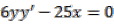represents a

Production And Industrial Engineering - PI 2015 GATE Paper (Practice Test) - Question 37

The solution to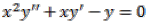is

Production And Industrial Engineering - PI 2015 GATE Paper (Practice Test) - Question 38

Match the linear transformation matrices listed in the first column to their interpretations in the second column

Production And Industrial Engineering - PI 2015 GATE Paper (Practice Test) - Question 39

The value of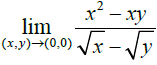is

Production And Industrial Engineering - PI 2015 GATE Paper (Practice Test) - Question 40

The curve y = x4 is

*Answer can only contain numeric values
Production And Industrial Engineering - PI 2015 GATE Paper (Practice Test) - Question 41

A metallic bar of uniform cross-section with specific weight of 100 kN/m3 is hung vertically down. The length and Young’s modulus of the bar are 100 m and 200 GPa, respectively. The elongation of the bar, in mm, due to its own weight is ____________.

(Important - Enter only the numerical value in the answer)

*Answer can only contain numeric values
Production And Industrial Engineering - PI 2015 GATE Paper (Practice Test) - Question 42

A beam is loaded as shown in the figure.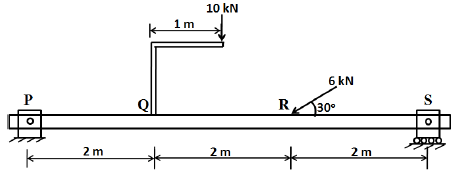The bending moment, in Nm, at point R is __________.

(Important - Enter only the numerical value in the answer)

Production And Industrial Engineering - PI 2015 GATE Paper (Practice Test) - Question 43

In an off-set slider crank mechanism, shown in figure, the crank is rotated at a constant speed of 150 rpm. The value of the angle θ shown in the figure is 200. What is the ratio of forward to return stroke time? Can this mechanism be used in an application involving quick return?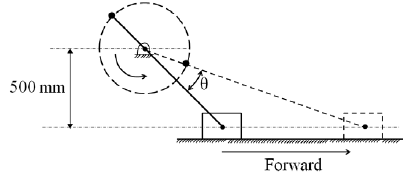*Answer can only contain numeric values
Production And Industrial Engineering - PI 2015 GATE Paper (Practice Test) - Question 44

In a 1 m thick wall, the temperature distribution at a given instant is T(x) = c0 + c1x + c2x2 where T is in oC and x is in m. The constants are: c0 = 800 oC, c1 = –250 oC/m and c2 = –40 oC/m2. The thermal conductivity of the wall is 50 W/mK and wall area is 5 m2. If there is a heat source generating uniform volumetric heating at the rate of 500 W/m3 inside the wall, then the rate of change of energy storage in the wall, in kW, is ________.

(Important - Enter only the numerical value in the answer)

Production And Industrial Engineering - PI 2015 GATE Paper (Practice Test) - Question 45

In a vertical piston-cylinder arrangement the force applied to the piston, pushes water through a nozzle as shown in the figure. The water flows out from the nozzle, and reaches the top of its trajectory. The kinetic and pressure energies at points (1), (2) and (3), respectively, are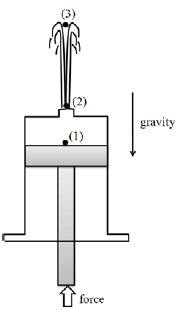Production And Industrial Engineering - PI 2015 GATE Paper (Practice Test) - Question 46

Consider a glass-fiber reinforced polymer material. The stress-strain curves of the fiber, matrix and composite are plotted in the figure. Which one of the following statements is TRUE?*Answer can only contain numeric values
Production And Industrial Engineering - PI 2015 GATE Paper (Practice Test) - Question 47

A mould for injection moulding is designed for polymer P having shrinkage of 0.010 mm/mm. A critical dimension needed in the moulded part is 35 mm. If the same mould is now used to make a similar part but made of a different polymer Q with shrinkage of 0.025 mm/mm, then the critical dimension in the moulded part made of polymer Q, in mm, is _____________.

(Important - Enter only the numerical value in the answer)

*Answer can only contain numeric values
Production And Industrial Engineering - PI 2015 GATE Paper (Practice Test) - Question 48

Open die forging of a cylinder made of a rigid perfectly plastic material with yield strength of 200 MPa having a height of 25 mm and diameter of 25 mm is being carried out. The cylinder is subjected to a true compressive strain of 3.6 during the process. Assuming frictionless and homogeneous deformation, the energy expended, in kJ, is _____________________.

(Important - Enter only the numerical value in the answer)

*Answer can only contain numeric values
Production And Industrial Engineering - PI 2015 GATE Paper (Practice Test) - Question 49

In drilling operation, a twist-drill of 30 mm diameter with point angle of 118 degrees is used. If the CNC command issued to execute the drilling operation is G90 G01 Z?? F20. The datum is defined on the top surface of the work material and the approach distance is 3 mm. Then, to achieve a cylindrical hole depth of 40 mm, the Z coordinate to be provided in the CNC command, in mm, is ________________.

(Important - Enter only the numerical value in the answer)

*Answer can only contain numeric values
Production And Industrial Engineering - PI 2015 GATE Paper (Practice Test) - Question 50

In an orthogonal machining experiment carried out using a cutting tool with zero degree rake angle, the measured cutting force was 1700 N. If the friction angle at the rake face-chip interface is 26o, then the thrust force value, in N, is _________________.

(Important - Enter only the numerical value in the answer)

*Answer can only contain numeric values
Production And Industrial Engineering - PI 2015 GATE Paper (Practice Test) - Question 51

In a slab milling operation, a cutter of 75 mm diameter with sufficient width is used to remove 5 mm thick material from a 200 mm long part in a single pass. The minimum length of travel, in mm, for the cutter to engage and completely cut the part surface is ___________.

(Important - Enter only the numerical value in the answer)

*Answer can only contain numeric values
Production And Industrial Engineering - PI 2015 GATE Paper (Practice Test) - Question 52

In a metal casting process, molten copper alloy is poured into a sand mould. The level of molten metal in the pouring basin is at a height of 300 mm from the runner having diameter of 10 mm. If the density and melting temperature of molten copper alloy are 9000 kg/m3 and 1000oC, respectively, then the rate of flow of molten metal into the mould neglecting friction and other losses, in cm3/s, is ____________________.

(Important - Enter only the numerical value in the answer)

Production And Industrial Engineering - PI 2015 GATE Paper (Practice Test) - Question 53

Two aluminum alloy plates each 10 mm thick and 1 m long are welded without crowning by multi-pass tungsten inert gas butt welding. The joint configuration is V-type with 60º angle and root gap is maintained at 5 mm. If electrode of 5 mm diameter with 500 mm length is used for welding, then the number of electrodes required is

*Answer can only contain numeric values
Production And Industrial Engineering - PI 2015 GATE Paper (Practice Test) - Question 54

A surface is prepared specially for an application with the profile as shown in the figure.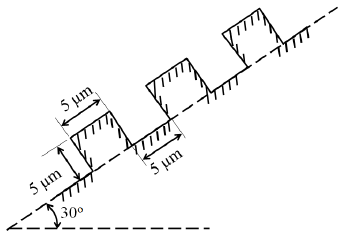The theoretical Ra value for this surface, in μm, is

(Important - Enter only the numerical value in the answer)

*Answer can only contain numeric values
Production And Industrial Engineering - PI 2015 GATE Paper (Practice Test) - Question 55

During the measurement of internal taper of a part using standard balls of diameter 15 mm and 20 mm, the large ball is found to protrude by 5 mm (h1) and the top of small ball is found to be 35 mm (h2) below the top face of the gauge. The taper angle, in degree, is ________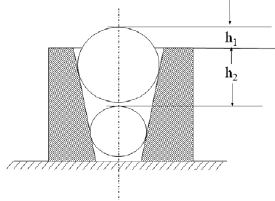(Important - Enter only the numerical value in the answer)

*Answer can only contain numeric values
Production And Industrial Engineering - PI 2015 GATE Paper (Practice Test) - Question 56

In a Flexible Manufacturing System, the Automated Guided Vehicles (AGV) move at a speed of 50 m/min, cover an average distance of 150 m to deliver and 100 m for return. If the time required for pick-up and drop is 30 s each, neglecting idle times, then the number of AGVs required to meet the demand of 50 deliveries per hour is ______________.

(Important - Enter only the numerical value in the answer)

*Answer can only contain numeric values
Production And Industrial Engineering - PI 2015 GATE Paper (Practice Test) - Question 57

A machine is bought for Rs. 25,00,000. The organization follows a declining balance method of depreciation with a depreciation charge of 25%. If the machine is sold at Rs.17,50,000 at the end of second year, then the profit on the book, in Rs., is ________________.

(Important - Enter only the numerical value in the answer)

*Answer can only contain numeric values
Production And Industrial Engineering - PI 2015 GATE Paper (Practice Test) - Question 58

A manufacturing line requires 7.2 minutes to make a product. The line has six workstations arranged as per the required sequence of operations. Total production required is 300 products in 7.5 hours. At steady state, the line efficiency, in %, is ____________.

(Important - Enter only the numerical value in the answer)

Production And Industrial Engineering - PI 2015 GATE Paper (Practice Test) - Question 59

A single facility is to be located to meet the demand at coordinates (1, 2), (2, 3), (3, 5) and (4, 1). The demand at these points is 700, 100, 300 and 500 respectively. Using the rectilinear distance measure and weighted distance minimization criterion, the facility should be located

Production And Industrial Engineering - PI 2015 GATE Paper (Practice Test) - Question 60

The value of (X1 , X2) for an optimal solution for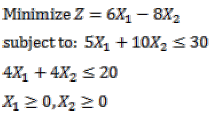is.

Production And Industrial Engineering - PI 2015 GATE Paper (Practice Test) - Question 61

Arrival of machines for repair in a maintenance shop follows a Poisson distribution at a rate of one per 18 hours. The time to repair follows an exponential distribution with Mean Time To Repair (MTTR) of 14 hours. If the productivity loss is Rs.22,500 per hour, then the total expected loss of productivity due to machine breakdowns, in Rs., is

Production And Industrial Engineering - PI 2015 GATE Paper (Practice Test) - Question 62

In a manufacturing process, 24 samples each of size 50 items were inspected and a total of 52 defective items were observed. The lower and upper control limits set for the p-chart should, respectively, be

*Answer can only contain numeric values
Production And Industrial Engineering - PI 2015 GATE Paper (Practice Test) - Question 63

Data on five products to be processed on a single machine is given below: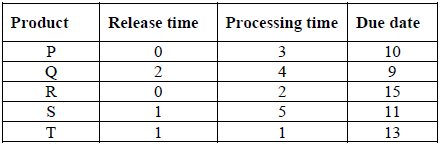For the processing sequence R – P – S – T – Q, total tardiness is ____________.

(Important - Enter only the numerical value in the answer)

*Answer can only contain numeric values
Production And Industrial Engineering - PI 2015 GATE Paper (Practice Test) - Question 64

In a time study experiment, observed time is 15 minutes, operator rating is 90, personal need allowance is 4%, fatigue allowance is 3%, contingency allowance for work is 3% and contingency allowance for delay is 2%. The total work content, in minutes, is __________________.

(Important - Enter only the numerical value in the answer)

Production And Industrial Engineering - PI 2015 GATE Paper (Practice Test) - Question 65

There are three alternatives to meet the demand of a product.

Alternative I: Manufacture using a process P

Alternative II: Manufacture using a process Q

Alternative III: Buy the product from a vendor

The costs associated with each alternative is given below: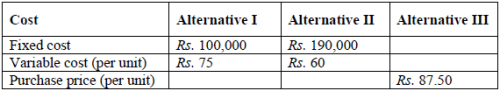Alternative I is cheaper compared to Alternative II when the demand is

## GATE Past Year Papers for Practice (All Branches)

407 docs|127 tests
Information about Production And Industrial Engineering - PI 2015 GATE Paper (Practice Test) Page
In this test you can find the Exam questions for Production And Industrial Engineering - PI 2015 GATE Paper (Practice Test) solved & explained in the simplest way possible. Besides giving Questions and answers for Production And Industrial Engineering - PI 2015 GATE Paper (Practice Test), EduRev gives you an ample number of Online tests for practice

## GATE Past Year Papers for Practice (All Branches)

407 docs|127 tests(Scan QR code)